# Division + area - math problems

#### Number of problems found: 61

• ParallogramThe Parallelogram base is 24 cm and high 10 cm. How many are such tiles required to cover a floor of area 1080m2?
• Rewrite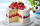Rewrite the following as division statement  if there are 8 cakes and each person get 1/12 of a cake how many people get cake?
• A piece 3A piece of rectangle cloth has an area of 2 4/5 meters. Its breadth is 1 1/5 meters what is the length of the cloth?
• Cups of paintI have a board that is 2 meters wide and 3 meters tall. If one cup of paint cover 1,000 square centimeters. How many cups of paints does I need?
• The rugJosie has a rug with the area of 18 square feet. She will put the rug on the floor that is covered in 1/3 square foot tiles. How many tiles will the rug cover?
• A pizza 2A pizza is cut into slices that are each 1/6 of the whole. John is going to eat 1/2 of the whole pizza. How many slices will John eat?
• The conical roof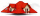The conical roof above the warehouse has a diameter of the lower part (base) d = 11.2 m and a height v = 3.3 m. How many rectangular steel plates with dimensions of 1.4 m and 0.9 m were needed for the production of this roof, if the seams and waste requir
• Divide an isosceles triangleHow to divide an isosceles triangle into two parts with equal contents perpendicular to the axis of symmetry (into a trapezoid and a triangle)?
• Cloth / textileWe have cloth measure 16 square meters. How many 20 cm by 20 cm by 8 cm bags you can make? Assume bag is a cuboid without one top base.
• Liliana's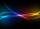Liliana's living room measures exactly 202.5 square feet. How many square yards is Liliana's living room?
• Roll of wallpaperAn art student uses a roll of wallpaper to decorate two gift boxes. The student will use 3 1/3 yards of paper for one box and 5/6 yard of paper for the other box. The paper must be cut into pieces that are 1/6 yard long. How many pieces will the student c
• Square gardensThe gardening colony with dimensions of 180 m and 300 m is to be completely divided into equally large square areas with the largest possible area. Calculate how many such square areas can be obtained and determine the side length of the square.
• The corridor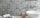The corridor is 12 m long and 3.6 m wide. It must be paved with rectangular tiles measuring 15 cm and 30 cm. Are 1000 pieces of tiles enough to pave the corridor?
• Two sides paintThe door has the shape of a rectangle with dimensions of 260cm and 170cm. How many cans of paint will be needed to paint this door if one can of paint cover 2m2 of the area? We paint the doors on both sides.
• Largest squaresHow many of the largest square sheets did the plumber cut the honeycomb from 16 dm and 96 dm?
• Tiles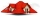The pupils of the building school have to calculate how many roof tiles they will need to cover the roof of the house in the shape of two rectangles measuring 10 x 7.2 m. One roof tile covers a rectangular area of 22 cmx32 cm. How many tiles will they nee
• Playground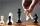On the special playground, there are 81 square sectors, each with a side of 5 m. How many players can fit on the playground if each player needs a 75 m2 area to play?
• The cylindrical container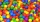The cylindrical container has a base area of 300 cm3 and a height of 10 cm. It is 90% filled with water. We gradually insert metal balls into the water, each with a volume of 20 cm3. After inserting how many balls for the first time does water flow over
• Paper cut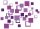How many 9 cm2 figures can we cut from 36 dm2 paper?
• Two bodiesThe rectangle with dimensions 8 cm and 4 cm is rotated 360º first around the longer side to form the first body. Then, we similarly rotate the rectangle around the shorter side b to form a second body. Determine the ratio of surfaces of the first and seco

Do you have an exciting math question or word problem that you can't solve? Ask a question or post a math problem, and we can try to solve it.

We will send a solution to your e-mail address. Solved examples are also published here. Please enter the e-mail correctly and check whether you don't have a full mailbox.

Please do not submit problems from current active competitions such as Mathematical Olympiad, correspondence seminars etc...

Division Problems. Area - math problems.